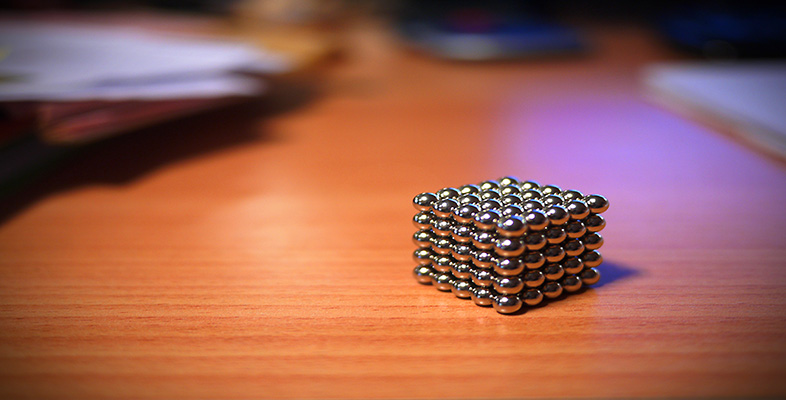Squares, roots and powers

This free course is available to start right now. Review the full course description and key learning outcomes and create an account and enrol if you want a free statement of participation.

Free course

1.4 Cubes

To find the cube of a number, multiply three copies of it together. For example: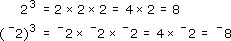You can use your calculator to find cubes. 23 is ‘two cubed’ or ‘two to the power three’. Just as ‘square root’ is the opposite process to squaring, so 'cube root' is the opposite process to cubing.

43 = 64, so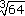= 4.

In the same way that square units are used to measure area, cubic units are used to measure volume. A cube measuring 1 cm × 1 cm × 1 cm has a volume of 1 centimetre cubed, or 1 cubic centimetre, written as 1 cm3, or 1 cc. The volume of a ‘box’ is length x width x height, so the volume of this cube (10 mm × 10 mm × 10 mm) in millimetres is

1 cm3 = 10 mm × 10 mm × 10 mm = 1000 mm3.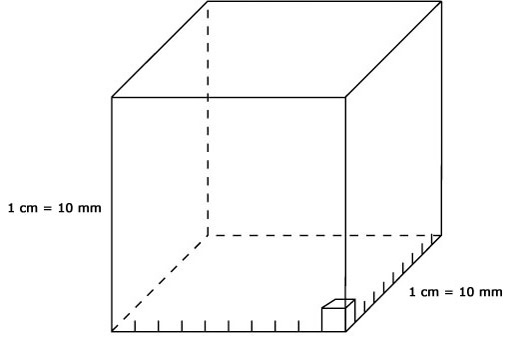Example 5

My half-litre measuring jug is marked off in divisions of 100 ml, with subdivisions of 20 ml. I want to measure out 420 cc, but the only conversion table I have tells me that a litre is one cubic decimetre. (One decimetre is a tenth of a metre.) How many cubic centimetres are there in a litre? Can I use the measuring jug for 420 cc?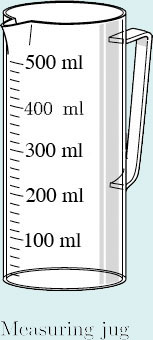There is one cubic decimetre in a litre, so 1 litre measures 1 dm × 1 dm × 1 dm (if shaped into a cube). (Note: 1 m = 10 dm = 100 cm.) There are 10 centimetres in a decimetre, so a 1 litre cube would measure

10 cm × 10 cm × 10 cm = 1000 cm3.

So 1 litre is 1000 cm3. This is a useful result.

But there are 1000 millilitres in a litre, so it turns out that a millilitre is the same as a cubic centimetre.

1 ml = 1 cm3.

So I can use the jug to measure 420 ml, or 420 cc (or cm3).

(Note: 1 cubic centimetre can be written as 1 cc or 1 cm3.)

MU120_4M4